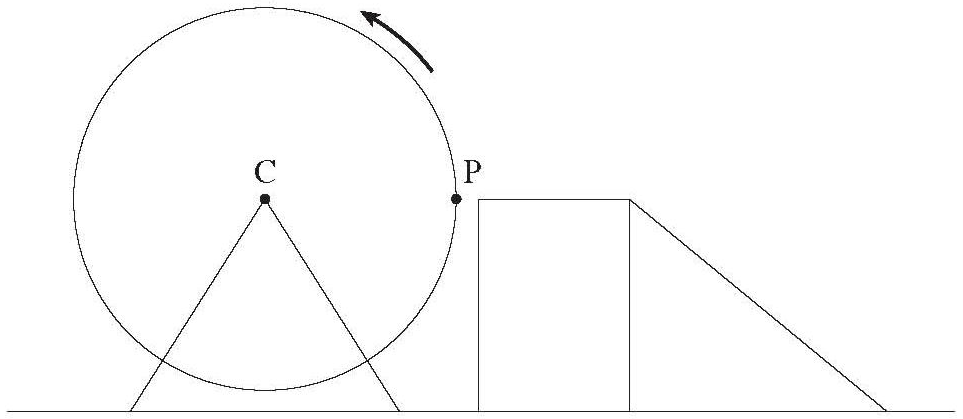# Ferris wheel trig problems

### Ferris wheel trig problems

#### Lessons

• 1.
A Ferris wheel has a radius of 18 meters and a center C which is 20m above the ground. It rotates once every 32 seconds in the direction shown in the diagram. A platform allows a passenger to get on the Ferris wheel at a point P which is 20m above the ground. If the ride begins at point P, when the time t = 0 seconds:a)
Graph how the height h of a passenger varies with respect to the elapsed time t during one rotation of the Ferris wheel. Clearly show at least 5 points on the graph.

b)
Determine a sinusoidal function that gives the passenger's height, h, in meters, above the ground as a function of time t seconds.

c)
How high above the ground would a passenger be 18 seconds after the Ferris wheel starts moving?

d)
How many seconds on each rotation is a passenger more than 30m in the air?